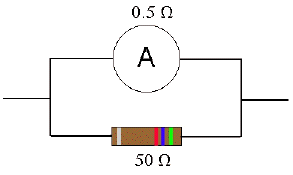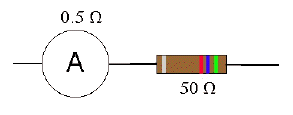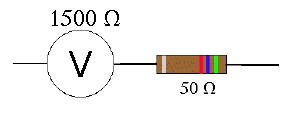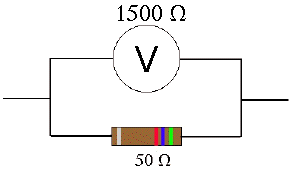Teaching Physics with the Physics Suite Edward F. Redish Problems Sorted by Type | Problems Sorted by Subject | Problems Sorted by Chapter in UP

Thinking about an ammeter and a voltmeter

An ammeter is an instrument that shows how much current passes through it. It is designed to have a small resistance. The following problems show why.

 A. Suppose an ammeter having a resistance of 0.5 Ω is connected in parallel across a resistor of 50.0 Ω as shown in the figure at the right. If the system is connected up to a 12 V battery, how much current would pass through the ammeter? (Assume you can ignore the internal resistance of the battery.) How much current would pass through the 50 ohm resistor?   Is this ammeter giving a good measure of the current in the 50 ohm resistor? Explain why or why not. Is this ammeter giving a good measurement of what the current would be in the resistor if the ammeter weren't there?B. Suppose instead the ammeter were in series with the resistor as shown in the figure at the right. If the system is connected up to a 12 V battery, how much current would pass through the meter? How much current would pass through the 50 ohm resistor?   Is this ammeter giving a good measure of the current in the 50 ohm resistor? Explain why or why not. Is this ammeter giving a good measurement of what the current would be in the resistor if the ammeter weren't there?A voltmeter is also a device that shows the voltage difference across its terminals, but it has a very large resistance. The next two problems show why.

 C. Suppose a voltmeter having a resistance of 1500 Ω were in series with a resistor of 50.0 Ω as shown in the figure at the right. If the system is connected up to a 12 V battery, what would the voltage drop be across the voltmeter? What would the voltage drop be across the 50 ohm resistor? Is this voltmeter giving a good measure of the voltage drop across the 50 ohm resistor? Explain why or why not. Is this voltmeter giving a good measurement of what the voltage drop across the resistor would be if the voltmeter weren't there?D. Suppose instead, the voltmeter were in parallel with the resistor as shown in the figure at the right. If the system is connected up to a 12 V battery, what would the voltage drop be across the meter? (Assume you can ignore the internal resistance of the battery.) What is the voltage drop across the 50 ohm resistor?   Is this voltmeter giving a good measure of the voltage drop across the 50 ohm resistor? Explain why or why not. Is this voltmeter giving a good measurement of what the voltage would be across the resistor if the voltmeter weren't there?E. So if you were to hook up an ammeter or a voltmeter to measure the current through a resistance or the voltage drop across a resistance respectively, how would you connect it?